Next: Propagazioni di incertezza, approssimazioni Up: Effetti sistematici e di Previous: Effetti sistematici e di   Indice

# Effetto del background nella misura dell'intensità di un processo di Poisson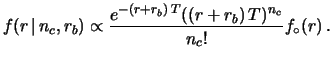(14.1)

and, making use of Bayes' theorem, we get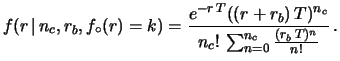(14.2)(14.3)

• A uniform distribution between 0 and 30: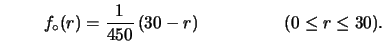(14.4)

• A triangular distribution: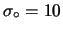(14.5)

• A half-Gaussian distribution of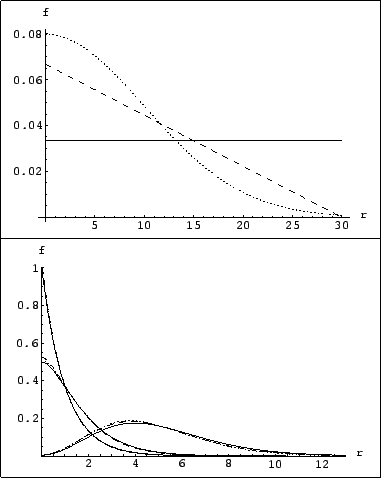(14.6)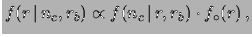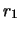(14.7)

and consider only two possible values of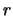, let them be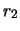and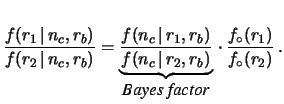. From (14.7) it follows that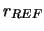(14.8)

The Bayes factor can be extended to a continuous set of hypotheses, considering a function which gives the Bayes factor of each value ofwith respect to a reference value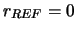. The reference value could be arbitrary, but for our problem the choice, giving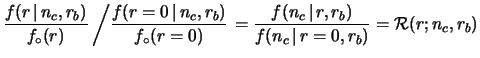(14.9)

is very convenient for comparing and combining the experimental results [#!ci!#,#!zeus!#,#!higgs!#]. The functionhas nice intuitive interpretations which can be highlighted by reordering the terms of (14.8) in the form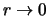(14.10)

(valid for all possible a priorivalues).has the probabilistic interpretation of relative belief updating ratio, or the geometrical interpretation of shape distortion function of the probability density function.goes to 1 for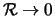, i.e. in the asymptotic region in which the experimental sensitivity is lost: As long as it is 1, the shape of the p.d.f. (and therefore the relative probabilities in that region) remains unchanged. Instead, in the limit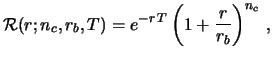(for large) the final p.d.f. vanishes, i.e. the beliefs go to zero no matter how strong they were before. In the case of the Poisson process we are considering, the relative belief updating factor becomes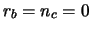(14.11)

with the condition14.1if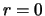.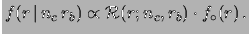These curves transmit the result of the experiment immediately and intuitively:
• whatever one's beliefs onwere before the data, these curves show how one must14.2 change them;
• the beliefs one had for rates far above 20 events/month are killed by the experimental result;
• if one believed strongly that the rate had to be below 0.1 events/month, the data are irrelevant;
• the case in which no candidate events have been observed gives the strongest constraint on the rate;
• the case of five candidate events over an expected background of one produces a peak ofwhich corroborates the beliefs around 4 events/month only if there were sizable prior beliefs in that region.
Moreover there are some technical advantages in reporting thefunction as a result of a search experiment.
• One deals with numerical values which can differ from unity only by a few orders of magnitude in the region of interest, while the values of the likelihood can be extremely low. For this reason, the comparison between different results given by thefunction can be perceived better than if these results were published in terms of likelihood.
• Sincediffers from the likelihood only by a factor, it can be used directly in Bayes' theorem, which does not depend on constants, whenever probabilistic considerations are needed.14.3In fact,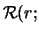(14.12)

• The combination of different independent results on the same14.4 quantitycan be done straightforwardly by multiplying individualfunctions: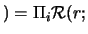all data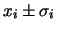data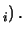(14.13)

• Finally, one does not need to decide a priori if one wants to make a discovery' or an upper limit' analysis as conventional statistics teaches (see e.g. criticisms in Ref. [#!BB!#]): thefunction represents the most unbiased way of presenting the results and everyone can draw their own conclusions.Next: Propagazioni di incertezza, approssimazioni Up: Effetti sistematici e di Previous: Effetti sistematici e di   Indice
Giulio D'Agostini 2001-04-02